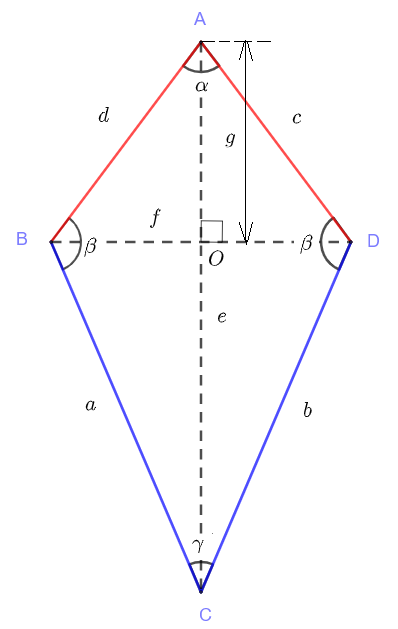# Kite Questions with Solutions

A kite is a quadrilateral with two pairs of adjacent sides equal in length. In the diagram below, sides $AB$ and $AD$ have equal lengths and sides $CB$ and $CD$ have equal lengths.
The diagonal axis $AC$ and the second diagonal $BD$ intersect at an angle of $90^{\circ}$## Kite Formulas

We define the length of segments $AC$, $BD$ and $AO$ using small letters as follows: $AC = e$, $BD = f$ and $AO = g$.
We now present the formulas that may be used to find the sides, the area, perimeter and angles of the kite.
Note that: $OC = AC - AO = e - g$ and $BO = OD = f/2$
Area of triangle $AOB = (1/2)(BO)(AO) = (1/4) f g$
Area of triangle $BOC = (1/2)(BO)(CO) = (1/4) f (e-g)$
Area of triangle $ABC = (1/4) f g + (1/4) f (e-g) = (1/4) f g$
Area $A$ of Kite: $\displaystyle A = 2 \times \text{Area of tringle} ABC = \dfrac{f \cdot e}{2}$
Use the Pythagorean theorem in the right triangle $BOC$
Sides $a$ and $b$: $\displaystyle a = b = \sqrt{ \left(\dfrac{f}{2}\right)^2 + (e-g)^2}$
Use the Pythagorean theorem in the right triangle $AOB$
Sides $c$ and $d$: $\displaystyle d = c = \sqrt{ \left(\dfrac{f}{2}\right)^2 + g^2}$
Perimeter: $\displaystyle p = 2 a + 2 d$
Use the right triangle $AOB$ to write: $\tan(\alpha / 2) = \dfrac{f/2}{g}$, which gives
Angle $\alpha$: $\displaystyle \alpha = 2 \arctan (\dfrac{f}{2g})$
Use the right triangle $BOC$ to write: $\tan(\gamma / 2) = \dfrac{f/2}{e-g}$, which gives
Angle$\gamma$ : $\displaystyle \gamma = 2 \arctan \left(\dfrac{f}{2(e-g)}\right)$
The sum of all angles in triangle $ABC$ is equal to $180^{\circ}$, hence
Angle $\beta$: $\displaystyle \beta = 180 - \dfrac{\gamma}{2} - \dfrac{\alpha}{2}$
A kite calculator is included and may be used to check answers to calculations.

## Questions with Solutions

Question 1
Calculate the sides $a$ and $d$, the area, the perimeter and the angles $\alpha, \beta$ and $\gamma$ of a kite with the diagonal axis of $0.8$ meters, the second diagonal $0.40$ meters and distance $AO$ of $0.2$ meters.
Solution
Given: $e = 0.8$, $f = 0.4$ and $g = 0.2$
Area: $\displaystyle A = \dfrac{f \cdot e}{2} = \dfrac{0.4 \cdot 0.8}{2} = 0.16$ square meters
Sides: $\displaystyle a = b = \sqrt{ \left(\dfrac{f}{2}\right)^2 + (e-g)^2} = \sqrt{ \left(\dfrac{0.4}{2}\right)^2 + (0.8-0.2)^2} \approx 0.63$ meters
Sides: $\displaystyle d = c = \sqrt{ \left(\dfrac{f}{2}\right)^2 + g^2} = \sqrt{ \left(\dfrac{0.4}{2}\right)^2 + 0.2^2} \approx 0.28$ meters
Perimeter: $\displaystyle p = 2 a + 2 d = 2 \cdot 0.68 + 2 \cdot 0.28 \approx 1.92$ meters
Angle: $\displaystyle \alpha = 2 \arctan (\dfrac{f}{2g}) = 2 \arctan (\dfrac{0.4}{2\cdot0.2}) = 90^{\circ}$
Angle: $\displaystyle \gamma = 2 \arctan (\dfrac{f}{2(e-g)}) = 2 \arctan (\dfrac{0.4}{2(0.8-0.2)}) = 36.87^{\circ}$
Angle: $\displaystyle \beta = 180 - \dfrac{\gamma}{2} - \dfrac{\alpha}{2} = 180 - \dfrac{36.87}{2} - \dfrac{90}{2} = 116.57^{\circ}$

Question 2
Calculate the sides $a$ and $d$, the angles $\beta$, $\gamma$, the area, the perimeter of a kite with the diagonal axis of $e = 1.5$ meters, the second diagonal $f = 0.50$ meters and angle $\alpha = 30^{\circ}$.
Solution
Use triangle $AOB$ to write: $\sin(\alpha/2) = \dfrac{f/2}{d}$
The above gives: $d = \dfrac{f/2}{\sin(\alpha/2)} = \dfrac{0.5/2}{\sin(15^{\circ}} = 0.97$ meters
Use triangle $AOB$ to write: $\tan(\alpha/2) = \dfrac{f/2}{OA}$
The above gives: $g = OA = \dfrac{f/2}{\tan(\alpha/2)} = \dfrac{0.5/2}{\tan(15^{\circ}} = 0.933$ meters
Use triangle $BOC$ to write: $\tan(\gamma/2) = \dfrac{f/2}{AC-AO} = \dfrac{0.5/2}{1.5 - 0.933} = 0.44091$
The above gives: $\gamma = 2 \arctan(0.44091) = 47.59^{\circ}$
Side: $a = \sqrt { (1.5 - 0.933)^2 + (0.5/2)^2} = 0.63$ meters
Angle: $\displaystyle \beta = 180 - \dfrac{\gamma}{2} - \dfrac{\alpha}{2} = 180 - \dfrac{47.59}{2} - \dfrac{30}{2} = 141.21^{\circ}$
Perimeter: $\displaystyle p = 2 a + 2 d = 2 \cdot 0.63 + 2 \cdot 0.97 \approx 3.2$ meters
Area: $\displaystyle A = \dfrac{f \cdot e}{2} = \dfrac{0.5 \cdot 1.5}{2} = 0.375$ square meters

Question 3
Given the second diagonal $f = 0.60$ meters and angles $\alpha = 30^{\circ}$ and $\beta = 120^{\circ}$, calculate the lengths of the diagonal axis $AC = e$ the second diagonal $BD = f$ and angle $\gamma$
Solution
Use triangle $AOB$ to write: $\tan(\alpha/2) = \dfrac{f/2}{OA}$
Which gives: $OA = g = \dfrac{f/2}{\tan(\alpha/2)} = \dfrac{0.60/2}{\tan(15^{\circ})} = 1.12$ meters
Use triangle $ABC$ to write: $\alpha / 2 + \gamma /2 + \beta = 180^{\circ}$
Hence: $\gamma = 2(180 - \alpha / 2 - \beta) = 2(180 - 15 - 120) = 90^{\circ}$
Use triangle $BOC$ to write: $\tan(\gamma / 2) = \dfrac{BO}{OC}$
Which gives: $OC = e - g = \dfrac{BO}{\tan(\gamma / 2)} = \dfrac{0.6/2}{45^{\circ}} = 0.3$
Hence: $e = 0.38 + g = 0.3 + 1.12 = 1.42$ meters.